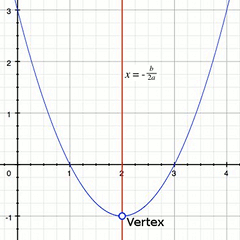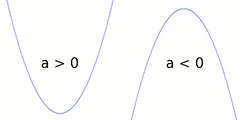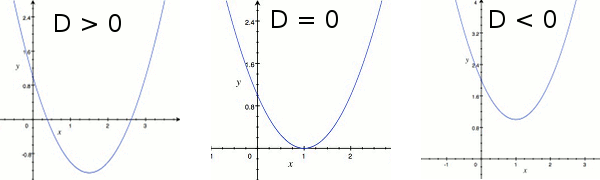A quadratic equation is a second degree polynomial equation. It has the general form:

$a{x}^{2}+bx+c=0\text{, with}\phantom{\rule{10px}{0ex}}a\ne 0$

The calculator below will calculate the Discriminant ($D$) and find the solutions (roots) of a quadratic equation.

Enter the values of the coefficients a, b, and c.

${x}^{2}$
$+$
$x$
$+$
$=0$

Please report any error to [email protected]

#### Properties of a quadratic equation:

•The graph of a quadratic equation is called a parabola.
• The axis of symmetry of the parabola is the line $x=\frac{-b}{2a}$ .
• The coordinate of the vertex of the parabola is found by evaluating $y$ at the axis of symmetry, i.e. evaluating $y$ at $x=\frac{-b}{2a}$ .
• If $a>0$ (i.e. positive), the parabola of the equation will face (open) up.
If $a<0$ (i.e. negative), the parabola of the equation will face (open) down.• The value of ${b}^{2}-4ac$ is called the Discriminant ($D$) of the quadratic equation.
By looking at the value of the discriminant of a quadratic equation, we can know the following:
• If the discriminant is positive ($D>0$), the quadratic equation has 2 distinct roots and both are real numbers. The parabola of the quadratic equation intercepts the x-axis at two points.
• If the discriminant is equal to zero ($D=0$), the quadratic equation has 1 root and it is a real number. The parabola of the quadratic equation intercepts the x-axis at exactly one point.
• If the discriminant is negative ($D<0$), the quadratic equation has 2 distinct roots and both are complex numbers. The parabola of the quadratic equation does not intercept the x-axis.#### Methods to solve or find the roots of a quadratic equation

There are a few methods to solve a quadratic equation, other than using the calculator above. The following are some of them.